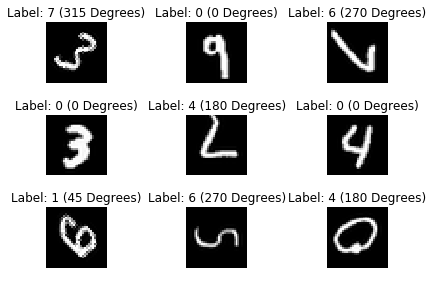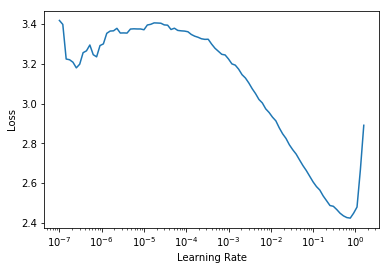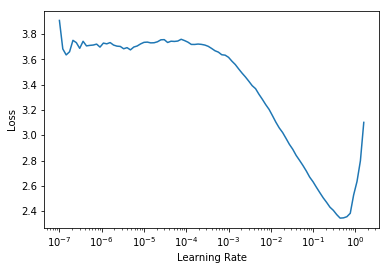# Introduction

This notebook is an introduction to self-supervised learning. In short, self-supervised learning has 2 components:

1. Pretrain on a pretext task, where the labels can come from the data itself!
2. Transfer the features, and train on the actual classification labels!

"What if we can get labels for free for unlabelled data and train unsupervised dataset in a supervised manner? We can achieve this by framing a supervised learning task in a special form to predict only a subset of information using the rest. In this way, all the information needed, both inputs and labels, has been provided. This is known as self-supervised learning." - Lilian Weng

Using FastAI2, we'll use rotation as a pretext task for learning representations/features of our data.

Here are some great overviews of self-supervised learning that I've come across:

# Experiment Layout

In this notebook, we will be using the MNIST dataset.

Also check out ImageWang from FastAI themselves! It's a dataset designed for self-supervision tasks!

1. Train a model on a rotation prediction task.

• We will use all the training data for rotation prediction.
• Input: A rotated image.
• Target/Label: Classify the amount of degrees rotated.
• Our model should learn useful features that can transfer well for a classification task.
• (The model should learn what digits look like in order to be able to successfully predict the amount of rotation).
2. Transfer our rotation pretraining features to solve the classification task with much fewer labels, < 1% of the original data.

• Input: A normal image.
• Target/Label: The images' original categorical label.
• Classification accuracy should be decent, even with only using < 1% of the original data.
3. Train a classifier from scratch on the same amount of data used in experiment 2.

• Input: A normal image.
• Target/Label: The images' original categorical label.
• Classification accuracy should be low (lack of transfer learning & too few labeled data!)
• Model may overfit.

# FastAI Vision Model Creation Methods

Warning: This Jupyter notebook runs with fastai2! Make sure you have it installed, use the cell below to install it :)
```!pip install fastai --upgrade

# Uncomment and run the below line to get a fresh install of fastai, if needed
```

Important: Pay attention! It’s important. We will be using a small ConvNet to test our self-supervised learning method. The architecture is defined below in `simple_arch`.
Note that `simple_arch` takes in one argument, `pretrained`. This is to allow FastAI to pass `pretrained=True` or `pretrained=False` when creating the model body! Below are some use cases of when we would want `pretrained=True` or `pretrained=False`.
1. `pretrained=False` = For training a new model on our rotation prediction task.
2. `pretrained=True` = For transferring the learnt features from our rotation task pretraining to solve a classification task.
3. `pretrained=False` = For training a new model from scratch on the main classification task (no transfer learning).
```from fastai.vision.all import *
```

```def simple_arch(pretrained=False):
# Note that FastAI will automatically cut at pooling layer for the body!
model = nn.Sequential(
nn.Conv2d(1, 4, 3, 1),
nn.BatchNorm2d(4),
nn.ReLU(),
nn.Conv2d(4, 16, 3, 1),
nn.BatchNorm2d(16),
nn.ReLU(),
nn.Conv2d(16, 32, 3, 1),
nn.BatchNorm2d(32),
)
if (pretrained):
return model
```

The follow below code snippets are examples of how FastAI creates CNNs. Every model will have a body and a head

```body = create_body(arch=simple_arch, pretrained=False)
body
```
```Sequential(
(0): Conv2d(1, 4, kernel_size=(3, 3), stride=(1, 1))
(1): BatchNorm2d(4, eps=1e-05, momentum=0.1, affine=True, track_running_stats=True)
(2): ReLU()
(3): Conv2d(4, 16, kernel_size=(3, 3), stride=(1, 1))
(4): BatchNorm2d(16, eps=1e-05, momentum=0.1, affine=True, track_running_stats=True)
(5): ReLU()
(6): Conv2d(16, 32, kernel_size=(3, 3), stride=(1, 1))
(7): BatchNorm2d(32, eps=1e-05, momentum=0.1, affine=True, track_running_stats=True)
)```

```head = create_head(nf=32, n_out=8, lin_ftrs=[])
```
```Sequential(
)
(1): Flatten(full=False)
(2): BatchNorm1d(64, eps=1e-05, momentum=0.1, affine=True, track_running_stats=True)
(3): Dropout(p=0.5, inplace=False)
(4): Linear(in_features=64, out_features=8, bias=False)
)```

```# Note that FastAI automatically determines nf for the head!
model = create_cnn_model(arch=simple_arch, pretrained=False, n_out=8, lin_ftrs=[])
model
```
```Sequential(
(0): Sequential(
(0): Conv2d(1, 4, kernel_size=(3, 3), stride=(1, 1))
(1): BatchNorm2d(4, eps=1e-05, momentum=0.1, affine=True, track_running_stats=True)
(2): ReLU()
(3): Conv2d(4, 16, kernel_size=(3, 3), stride=(1, 1))
(4): BatchNorm2d(16, eps=1e-05, momentum=0.1, affine=True, track_running_stats=True)
(5): ReLU()
(6): Conv2d(16, 32, kernel_size=(3, 3), stride=(1, 1))
(7): BatchNorm2d(32, eps=1e-05, momentum=0.1, affine=True, track_running_stats=True)
)
(1): Sequential(
)
(1): Flatten(full=False)
(2): BatchNorm1d(64, eps=1e-05, momentum=0.1, affine=True, track_running_stats=True)
(3): Dropout(p=0.5, inplace=False)
(4): Linear(in_features=64, out_features=8, bias=False)
)
)```

# PyTorch Rotation/Classification Self-Supervised Dataset

```import torchvision
tensorToImage = torchvision.transforms.ToPILImage()
imageToTensor = torchvision.transforms.ToTensor()
```

```# Uncomment and run the below lines if torchvision has trouble downloading MNIST (in the next cell)

# !wget -P data/MNIST/raw/ http://yann.lecun.com/exdb/mnist/train-images-idx3-ubyte.gz
# !wget -P data/MNIST/raw/ http://yann.lecun.com/exdb/mnist/train-labels-idx1-ubyte.gz
# !wget -P data/MNIST/raw/ http://yann.lecun.com/exdb/mnist/t10k-images-idx3-ubyte.gz
# !wget -P data/MNIST/raw/ http://yann.lecun.com/exdb/mnist/t10k-labels-idx1-ubyte.gz
```
```torchvision.datasets.MNIST('data/', download=True)
```
```Dataset MNIST
Number of datapoints: 60000
Root location: data/
Split: Train```

Below we define a dataset, here's the docstring:

A Dataset for Rotation-based Self-Supervision! Images are rotated clockwise.

• `file` - MNIST processed .pt file.
• `pct` - percent of data to use
• `classification` - False=Use rotation labels. True=Use original classification labels.

```class Custom_Dataset_MNIST():
'''
A Dataset for Rotation-based Self-Supervision! Images are rotated clockwise.
- file - MNIST processed .pt file.
- pct - percent of data to use
- classification - False=Use rotation labels. True=Use original classification labels.
'''

def __init__(self, file, pct, classification):

self.imgs = data
self.labels = data
self.pct = pct
self.classification = classification

slice_idx = int(len(self.imgs)*self.pct)
self.imgs = self.imgs[:slice_idx]

def __len__(self):
return len(self.imgs)

def __getitem__(self, idx):
img = self.imgs[idx].unsqueeze(0)
img = tensorToImage(img)
img = img.resize((32, 32), resample=1)
img = imageToTensor(img)

if (not self.classification):
# 4 classes for rotation
degrees = [0, 45, 90, 135, 180, 225, 270, 315]
rand_choice = random.randint(0, len(degrees)-1)

img = tensorToImage(img)
img = img.rotate(degrees[rand_choice])
img = imageToTensor(img)
return img, torch.tensor(rand_choice).long()

return img, self.labels[idx]

def show_batch(self, n=3):
fig, axs = plt.subplots(n, n)
fig.tight_layout()
for i in range(n):
for j in range(n):
rand_idx = random.randint(0, len(self)-1)
img, label = self.__getitem__(rand_idx)
axs[i, j].imshow(tensorToImage(img), cmap='gray')
if self.classification:
axs[i, j].set_title('Label: {0} (Digit #{1})'.format(label.item(), label.item()))
else:
axs[i, j].set_title('Label: {0} ({1} Degrees)'.format(label.item(), label.item()*45))
axs[i, j].axis('off')
```

# Rotation Prediction Data

Important: 60k training data and 10k validation data!
```train_ds = Custom_Dataset_MNIST('data/MNIST/processed/training.pt', pct=1.0, classification=False)
valid_ds = Custom_Dataset_MNIST('data/MNIST/processed/test.pt', pct=1.0, classification=False)
print('{0} Training Samples | {1} Validation Samples'.format(len(train_ds), len(valid_ds)))
```
```60000 Training Samples | 10000 Validation Samples
```

Note: Notice that our labels don’t correspond to digits! They correspond to the amount of degrees rotated! Specifically from this predefined set: `[0, 45, 90, 135, 180, 225, 270, 315]`
```from fastai.data.core import DataLoaders

# Override the show_batch function of dls to the one used in our dataset!
dls.show_batch = train_ds.show_batch

# We have 8 classes! [0, 1, 2, 3, 4, 5, 6, 7] that correspond to the [0, 45, 90, 135, 180, 225, 270, 315] degrees of rotation.
dls.c = 8

dls.show_batch()
```# FastAI Vision Learner [Rotation]

```rotation_head = create_head(nf=32, n_out=8, lin_ftrs=[])
```
```Sequential(
)
(1): Flatten(full=False)
(2): BatchNorm1d(64, eps=1e-05, momentum=0.1, affine=True, track_running_stats=True)
(3): Dropout(p=0.5, inplace=False)
(4): Linear(in_features=64, out_features=8, bias=False)
)```

Note: We want to measure `top_2_accuracy` along with regular (top_1) accuracy, because there are hard-cases where it’s understandable why our model got it wrong. For example: ’0’ rotated 90 or 270 degrees, or ’1’ rotated 0 or 180 degrees. (They can look the same!)
```# - A zero rotated 90 or 270 degrees?
# - A one rotated 0 or 180 degrees?
# etc :P

top_2_accuracy = lambda inp, targ: top_k_accuracy(inp, targ, k=2)
top_2_accuracy
```
`<function __main__.<lambda>(inp, targ)>`

Here, we train a model on the rotation prediction task!

```# Note to set a value for lin_ftrs, we use the defined config above.
learner = cnn_learner(dls,
simple_arch,
pretrained=False,
loss_func=CrossEntropyLossFlat(),
metrics=[accuracy, top_2_accuracy])

learner.model
```
```Sequential(
(0): Sequential(
(0): Conv2d(1, 4, kernel_size=(3, 3), stride=(1, 1))
(1): BatchNorm2d(4, eps=1e-05, momentum=0.1, affine=True, track_running_stats=True)
(2): ReLU()
(3): Conv2d(4, 16, kernel_size=(3, 3), stride=(1, 1))
(4): BatchNorm2d(16, eps=1e-05, momentum=0.1, affine=True, track_running_stats=True)
(5): ReLU()
(6): Conv2d(16, 32, kernel_size=(3, 3), stride=(1, 1))
(7): BatchNorm2d(32, eps=1e-05, momentum=0.1, affine=True, track_running_stats=True)
)
(1): Sequential(
)
(1): Flatten(full=False)
(2): BatchNorm1d(64, eps=1e-05, momentum=0.1, affine=True, track_running_stats=True)
(3): Dropout(p=0.5, inplace=False)
(4): Linear(in_features=64, out_features=8, bias=False)
)
)```
```learner.summary()
```
```Sequential (Input shape: 64)
============================================================================
Layer (type)         Output Shape         Param #    Trainable
============================================================================
64 x 4 x 30 x 30
Conv2d                                    40         True
BatchNorm2d                               8          True
ReLU
____________________________________________________________________________
64 x 16 x 28 x 28
Conv2d                                    592        True
BatchNorm2d                               32         True
ReLU
____________________________________________________________________________
64 x 32 x 26 x 26
Conv2d                                    4640       True
BatchNorm2d                               64         True
____________________________________________________________________________
[]
Flatten
BatchNorm1d                               128        True
Dropout
____________________________________________________________________________
64 x 8
Linear                                    512        True
____________________________________________________________________________

Total params: 6,016
Total trainable params: 6,016
Total non-trainable params: 0

Optimizer used: <function Adam at 0x7fdc9da95840>
Loss function: FlattenedLoss of CrossEntropyLoss()

Callbacks:
- TrainEvalCallback
- Recorder
- ProgressCallback```
```learner.lr_find()
```
`SuggestedLRs(lr_min=0.07585775852203369, lr_steep=0.013182567432522774)````learner.fit_one_cycle(5, lr_max=3e-2)
```
</tr> </thead> </table> </div> </div> </div> </div> </div>

Important: We were able to achieve 76.2% top-1 accuracy, and 96.8% top-2 accuracy after just 5 epochs! Now we want to grab our `model` from our `Learner`, and save the body of it!

Note: Our `model` has two components, the body and the head. `model` is a list of size 2, where `model` is the body, and `model` is the head!
```trained_body = learner.model
trained_body
```
```Sequential(
(0): Conv2d(1, 4, kernel_size=(3, 3), stride=(1, 1))
(1): BatchNorm2d(4, eps=1e-05, momentum=0.1, affine=True, track_running_stats=True)
(2): ReLU()
(3): Conv2d(4, 16, kernel_size=(3, 3), stride=(1, 1))
(4): BatchNorm2d(16, eps=1e-05, momentum=0.1, affine=True, track_running_stats=True)
(5): ReLU()
(6): Conv2d(16, 32, kernel_size=(3, 3), stride=(1, 1))
(7): BatchNorm2d(32, eps=1e-05, momentum=0.1, affine=True, track_running_stats=True)
)```

Tip: To save a model in PyTorch, save it’s `state_dict` function! You can use `model.load_state_dict` to re-load the weights.
```save_path = Path('rotation_cps/')
if not save_path.exists():
save_path.mkdir()

# Save the rotation-pretraining weights of our model body
torch.save(trained_body.state_dict(), save_path/'rot_pretrained.pt')
```

# Original Classification Data

Now that we have pretrained our model on the rotation prediction task, we want to switch over to the original labeled data for the classification task.

Important: We’re only using 180 samples for training!
```# Use 100% classification labeled data for validation!
train_ds = Custom_Dataset_MNIST('data/MNIST/processed/training.pt', pct=0.003, classification=True)
valid_ds = Custom_Dataset_MNIST('data/MNIST/processed/test.pt', pct=1.0, classification=True)
print('{0} Training Samples | {1} Validation Samples'.format(len(train_ds), len(valid_ds)))
```
```180 Training Samples | 10000 Validation Samples
```

Note: Notice the labels now correspond to the digit class!
```from fastai.data.core import DataLoaders
dls.show_batch = train_ds.show_batch

# We have 10 classes! One for each digit label!
dls.c = 10

dls.show_batch()
```# FastAI Vision Learner [Transfer-Classification]

Here we will toggle `pretrained=True` to transfer our rotation prediction features, and train on the original 180 labeled data.

```classification_head = create_head(nf=32, n_out=10, lin_ftrs=[])
```
```Sequential(
)
(1): Flatten(full=False)
(2): BatchNorm1d(64, eps=1e-05, momentum=0.1, affine=True, track_running_stats=True)
(3): Dropout(p=0.5, inplace=False)
(4): Linear(in_features=64, out_features=10, bias=False)
)```

Note: We have `n_out=10` because of the 10 different digit classes

```# pretrained=True will load the saved rotation pretraining weights into our model's body!
# See simple_arch() function definition for more details!
learner = cnn_learner(dls,
simple_arch,
pretrained=True,
loss_func=CrossEntropyLossFlat(),
metrics=[accuracy])

learner.model
```
```Loading pretrained model...
<All keys matched successfully>
```
```Sequential(
(0): Sequential(
(0): Conv2d(1, 4, kernel_size=(3, 3), stride=(1, 1))
(1): BatchNorm2d(4, eps=1e-05, momentum=0.1, affine=True, track_running_stats=True)
(2): ReLU()
(3): Conv2d(4, 16, kernel_size=(3, 3), stride=(1, 1))
(4): BatchNorm2d(16, eps=1e-05, momentum=0.1, affine=True, track_running_stats=True)
(5): ReLU()
(6): Conv2d(16, 32, kernel_size=(3, 3), stride=(1, 1))
(7): BatchNorm2d(32, eps=1e-05, momentum=0.1, affine=True, track_running_stats=True)
)
(1): Sequential(
)
(1): Flatten(full=False)
(2): BatchNorm1d(64, eps=1e-05, momentum=0.1, affine=True, track_running_stats=True)
(3): Dropout(p=0.5, inplace=False)
(4): Linear(in_features=64, out_features=10, bias=False)
)
)```

Tip: Freezing a model’s body after transferring the weights over, allows the new head to get calibrated with the rest of the model!
```learner.freeze()
```

Note: Looking at the model summary, we can see that the model is frozen up to the new head! Good!
```learner.summary()
```
```Sequential (Input shape: 64)
============================================================================
Layer (type)         Output Shape         Param #    Trainable
============================================================================
64 x 4 x 30 x 30
Conv2d                                    40         False
BatchNorm2d                               8          True
ReLU
____________________________________________________________________________
64 x 16 x 28 x 28
Conv2d                                    592        False
BatchNorm2d                               32         True
ReLU
____________________________________________________________________________
64 x 32 x 26 x 26
Conv2d                                    4640       False
BatchNorm2d                               64         True
____________________________________________________________________________
[]
Flatten
BatchNorm1d                               128        True
Dropout
____________________________________________________________________________
64 x 10
Linear                                    640        True
____________________________________________________________________________

Total params: 6,144
Total trainable params: 872
Total non-trainable params: 5,272

Optimizer used: <function Adam at 0x7fdc9da95840>
Loss function: FlattenedLoss of CrossEntropyLoss()

Model frozen up to parameter group #1

Callbacks:
- TrainEvalCallback
- Recorder
- ProgressCallback```
```learner.lr_find()
```
`SuggestedLRs(lr_min=0.07585775852203369, lr_steep=0.14454397559165955)````learner.fit_one_cycle(10, lr_max=3e-2)
```
epoch train_loss valid_loss accuracy </th> time
0 0.932141 1.084204 0.502600 0.875300 00:12
1 0.861757 0.831546 0.631500 0.905800 00:12
2 0.757740 0.841575 0.612800 0.932800 00:12
3 0.678802 0.655642 0.706700 0.949000 00:11
4 0.629287 0.550476 0.762000 0.968400 00:12
epoch train_loss valid_loss accuracy time
0 3.752726 4.032210 0.113500 00:01
1 3.530574 3.048504 0.131500 00:01
2 3.269396 2.611858 0.103700 00:01
3 2.922121 2.407352 0.156700 00:01
4 2.598596 2.234617 0.183000 00:01
5 2.351149 2.088923 0.180100 00:01
6 2.157926 1.959662 0.199200 00:01
7 1.994265 1.835968 0.250600 00:01
8 1.874377 1.722400 0.319700 00:01
9 1.754826 1.629582 0.388200 00:01

Tip: Unfreeze the model after calibrating the new head with the transferred body, and train a little more!
```learner.unfreeze()
```
```learner.summary()
```
```Sequential (Input shape: 64)
============================================================================
Layer (type)         Output Shape         Param #    Trainable
============================================================================
64 x 4 x 30 x 30
Conv2d                                    40         True
BatchNorm2d                               8          True
ReLU
____________________________________________________________________________
64 x 16 x 28 x 28
Conv2d                                    592        True
BatchNorm2d                               32         True
ReLU
____________________________________________________________________________
64 x 32 x 26 x 26
Conv2d                                    4640       True
BatchNorm2d                               64         True
____________________________________________________________________________
[]
Flatten
BatchNorm1d                               128        True
Dropout
____________________________________________________________________________
64 x 10
Linear                                    640        True
____________________________________________________________________________

Total params: 6,144
Total trainable params: 6,144
Total non-trainable params: 0

Optimizer used: <function Adam at 0x7fdc9da95840>
Loss function: FlattenedLoss of CrossEntropyLoss()

Model unfrozen

Callbacks:
- TrainEvalCallback
- Recorder
- ProgressCallback```
```learner.lr_find()
```
`SuggestedLRs(lr_min=0.025118863582611083, lr_steep=6.309573450380412e-07)````learner.fine_tune(5, base_lr=3e-2)
```
epoch train_loss valid_loss accuracy time
0 0.926475 1.532705 0.473400 00:01
epoch train_loss valid_loss accuracy time
0 1.057154 1.468854 0.514800 00:01
1 1.079370 1.409364 0.542100 00:01
2 1.009147 1.357950 0.562100 00:01
3 0.976450 1.306930 0.586400 00:01
4 0.935184 1.269005 0.606100 00:01

Important: We were able to get 60.6% accuracy using transfer learning from our pretraining on the rotation prediction task!

# FastAI Vision Learner [From Sratch-Classification]

Here we train a model from scratch on the original 180 labeled data.

```# pretrained=False, Create the same model as before, but without using the rotation pretraining weights!
learner = cnn_learner(dls,
simple_arch,
pretrained=False,
loss_func=CrossEntropyLossFlat(),
metrics=[accuracy])

learner.model
```
```Sequential(
(0): Sequential(
(0): Conv2d(1, 4, kernel_size=(3, 3), stride=(1, 1))
(1): BatchNorm2d(4, eps=1e-05, momentum=0.1, affine=True, track_running_stats=True)
(2): ReLU()
(3): Conv2d(4, 16, kernel_size=(3, 3), stride=(1, 1))
(4): BatchNorm2d(16, eps=1e-05, momentum=0.1, affine=True, track_running_stats=True)
(5): ReLU()
(6): Conv2d(16, 32, kernel_size=(3, 3), stride=(1, 1))
(7): BatchNorm2d(32, eps=1e-05, momentum=0.1, affine=True, track_running_stats=True)
)
(1): Sequential(
)
(1): Flatten(full=False)
(2): BatchNorm1d(64, eps=1e-05, momentum=0.1, affine=True, track_running_stats=True)
(3): Dropout(p=0.5, inplace=False)
(4): Linear(in_features=64, out_features=10, bias=False)
)
)```
```learner.summary()
```
```Sequential (Input shape: 64)
============================================================================
Layer (type)         Output Shape         Param #    Trainable
============================================================================
64 x 4 x 30 x 30
Conv2d                                    40         True
BatchNorm2d                               8          True
ReLU
____________________________________________________________________________
64 x 16 x 28 x 28
Conv2d                                    592        True
BatchNorm2d                               32         True
ReLU
____________________________________________________________________________
64 x 32 x 26 x 26
Conv2d                                    4640       True
BatchNorm2d                               64         True
____________________________________________________________________________
[]
Flatten
BatchNorm1d                               128        True
Dropout
____________________________________________________________________________
64 x 10
Linear                                    640        True
____________________________________________________________________________

Total params: 6,144
Total trainable params: 6,144
Total non-trainable params: 0

Optimizer used: <function Adam at 0x7fdc9da95840>
Loss function: FlattenedLoss of CrossEntropyLoss()

Callbacks:
- TrainEvalCallback
- Recorder
- ProgressCallback```
```learner.lr_find()
```
`SuggestedLRs(lr_min=0.04365158379077912, lr_steep=0.010964781977236271)````learner.fit_one_cycle(10, lr_max=3e-2)
```
epoch train_loss valid_loss accuracy time
0 3.689067 6.550608 0.101000 00:01
1 3.290434 5.411524 0.101100 00:01
2 2.885511 4.980519 0.074900 00:01
3 2.584637 9.233224 0.102800 00:01
4 2.328669 12.973579 0.102800 00:01
5 2.115707 14.907648 0.102800 00:01
6 1.952985 15.155903 0.102800 00:01
7 1.831354 14.910644 0.102800 00:01
8 1.734552 14.626731 0.103000 00:01
9 1.643174 14.119630 0.103400 00:01

Important: We were able to only get 10.3% accuracy with training from scratch

# Conclusion

Important: Using self-supervision can help learn features that can transfer to a down-stream task, such as classification! In this example, we used rotation predication as our pretext task for feature representation learning. Pretraining our model on rotation prediction prior to training for classification allowed us to achieve 60.6% accuracy, on just 0.3% of the labeled data (180 samples)! Training from scratch with the same amount of data yields an accuracy of 10.3%. The motivation for using self-supervised learning is the ability to train models with decent accuracy without the need of much labeled data!

Note: Be sure to try other self-supervised learning methods (or perhaps find your own!) and compete on the ImageWang Leadboard! How will model size, data difficultly, and dataset size (number of samples) affect self-supervised learning?
</div>